# 计算机视觉大作业

## 基于Opencv+keras的手势识别系统

#### 技术

python3.6 + opencv + keras + numpy + PIL

### 图片处理

``````   # 图像边缘处理--腐蚀
# 将原始图像与腐蚀处理后的图片做"与"操作
# 灰度处理
gray = cv2.cvtColor(bitwise_and, cv2.COLOR_BGR2GRAY)
# 高斯滤波
blur = cv2.GaussianBlur(gray, (self.blurValue, self.blurValue), 2)
cv2.imshow('GaussianBlur', blur)
#图像阈值化的一般目的是从灰度图像中分享目标区域和背景区域
cv2.imshow('thresh', thresh)
Ges = cv2.resize(thresh, (100, 100))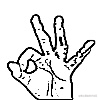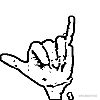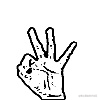### 数据增强

#### 数据增强作用

``````  test_datagen = ImageDataGenerator(rescale=1. / 255)
train_datagen = ImageDataGenerator(
rescale=1. / 255,
rotation_range=40,
width_shift_range=0.2,
height_shift_range=0.2,
shear_range=0.2,
zoom_range=0.2,
horizontal_flip=True)

train_dir = r'Gesture_predict'
validation_dir = r'Gesture_train'
# train_img_list, test_img_list, train_lable_list, test_lable_list
train_datagen.fit(train_img_list)
train_generator = train_datagen.flow(train_img_list,train_lable_list,batch_size=10)
validation_generator = test_datagen.flow(test_img_list,test_lable_list,batch_size=10)

### 特征提取

``````        def extarct_features(flag, sample_count):
features = np.zeros(shape=(sample_count, 3, 3, 512))
labels = np.zeros(shape=(sample_count,5))
if flag=="train":
generator = datagen.flow(train_img_list,train_lable_list,batch_size=20)
else:
generator = datagen.flow(test_img_list,test_lable_list,batch_size=20)
i = 0
for inputs_batch, labels_batch in generator:
if i * (batch_size+1 )>= sample_count:
break
features_batch = conv_base.predict(inputs_batch)
features[i * batch_size: (i + 1) * batch_size] = features_batch
labels[i * batch_size: (i + 1) * batch_size] = labels_batch
i += 1

return features, labels

### 模型改进

dropout：在每次训练的时候，让某些的特征检测器停过工作，即让神经元以一定的概率不被激活，这样可以防止过拟合，提高泛化能力

## 模型的结构图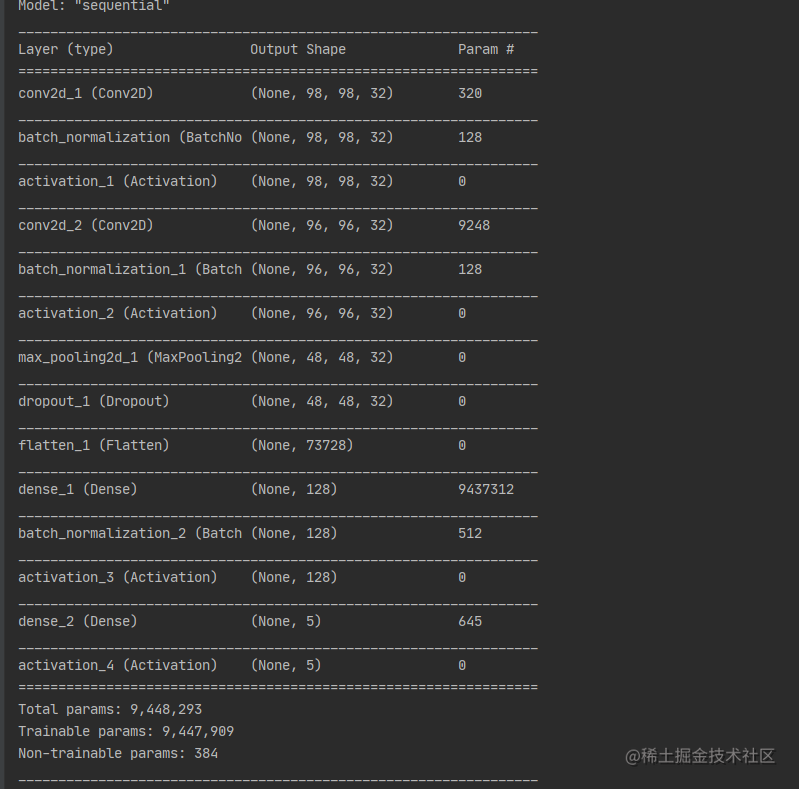`````` # 批规范化处理
# 激活函数relu
# 卷积层2，个数32，尺寸3*3，填充方式valid，步长默认1*1
filters=32,
kernel_size=(3, 3),
name='conv2d_2'
))

# 池化层，尺寸2*2，步长为2*2，填充方式为valid
pool_size=(2, 2),
strides=(2, 2),
name='max_pooling2d_1'
))
# dropout层，失活系数0.5
# 转化为一维矩阵
# 全连接层，128个神经元

# 分类层，L2正则优化
# kernel_regularizer=regularizers.l2(0.01),
name='dense_2'))
# 分类层，激活函数sofomax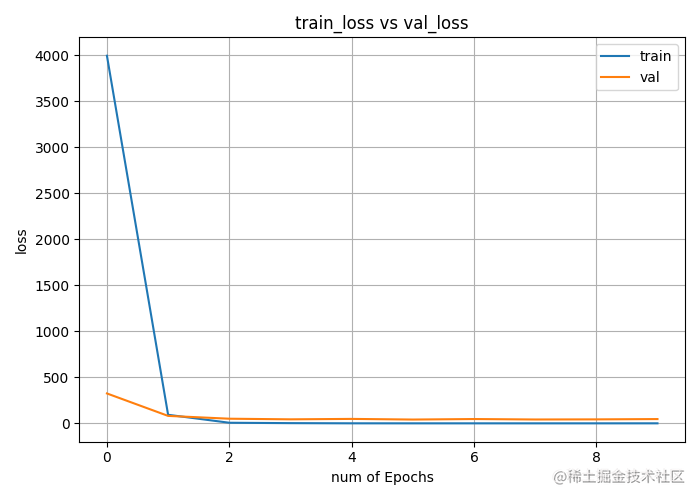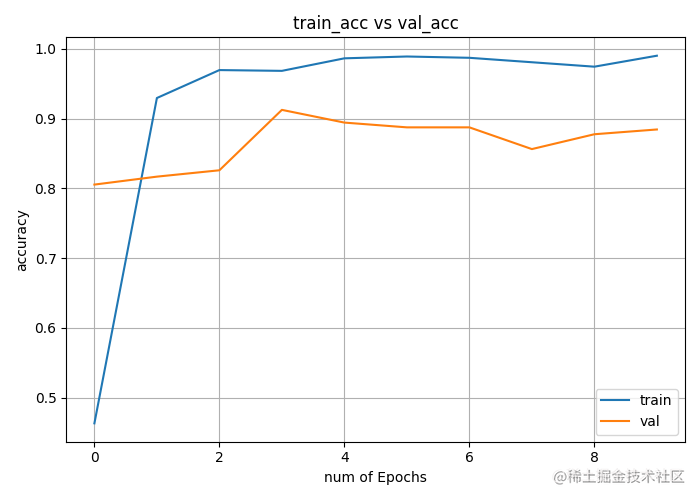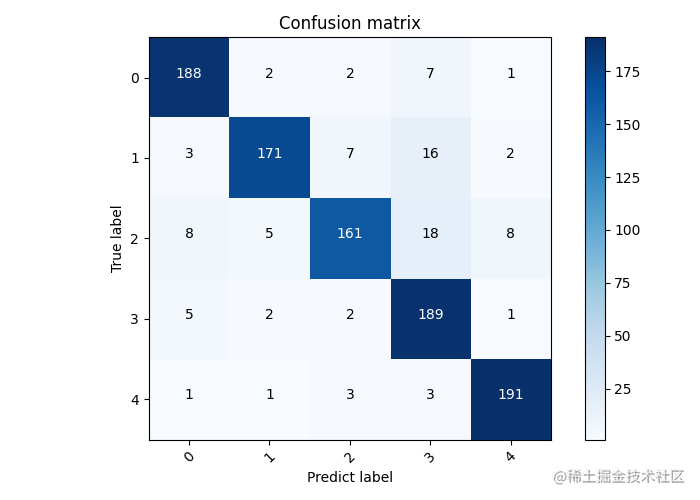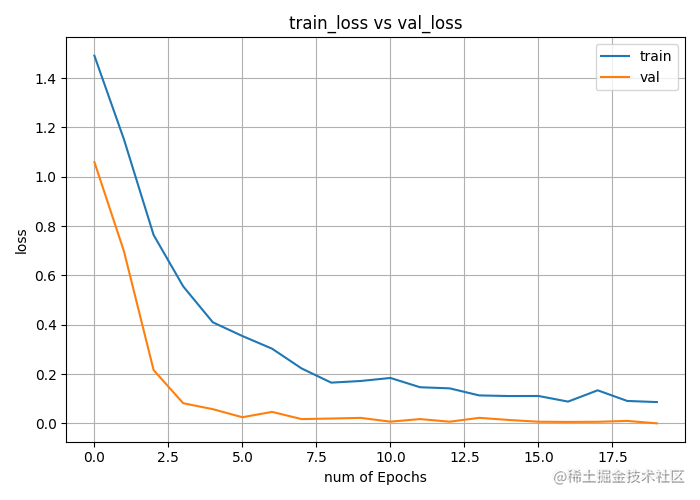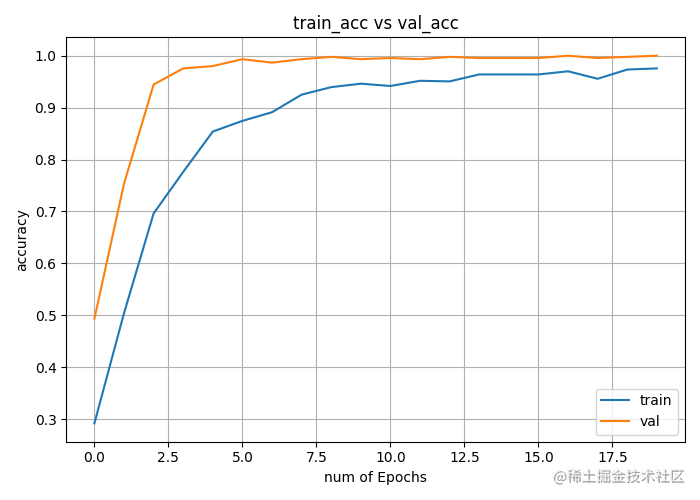# 稿子

1. 图片处理： 提取出照片的主体内容，去掉噪音和毕竟
2. 数据增强：提高数据泛化能力